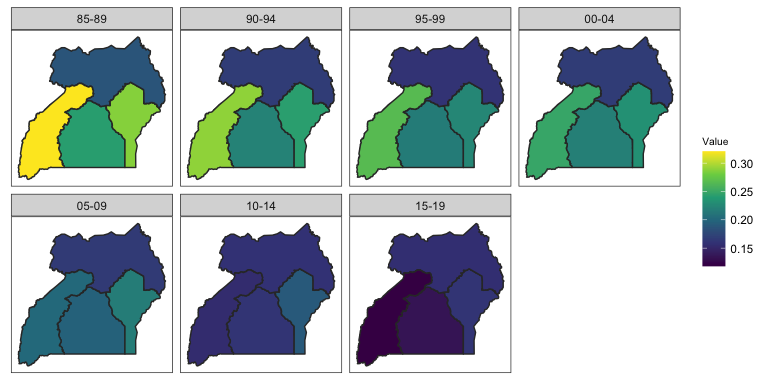# SUMMER: Bayesian Small Area Estimation using Complex Survey Data

#### 2020/07/02

The SUMMER package offers a class of tools for small-area estimation with survey data in space and time. Such data are usually collected with complex stratified designs, which must be acknowledged in the analysis. In this vignette, we use two examples to illustrate spatial and spatial-temporal smoothing of design-based estimates:

• Naive, spatial, and spatial-temporal smoothing of design-based estimates using BRFSS data.
• Spatial-temporal smoothing under-5 child mortality rates (U5MR) using DHS example data.

The first BRFSS example may be of more general interest, and provides an introduction to the idea of small area estimation with survey data. The second example of estimating U5MR involves more modeling steps to deal with the composite mortality indicator and data sparsity. More details of applying the methods to real world data are discussed in the package vignette A Case Study of Estimating Subnational U5MR using Smoothed Direct Methods.

## Small Area Estimation with BRFSS Data

The Behavioral Risk Factor Surveillance System (BRFSS) is an annual telephone health survey conducted by the Centers for Disease Control and Prevention (CDC) that tracks health conditions and risk behaviors in the United States and its territories since 1984. The BRFSS sampling scheme is complex with high variability in the sampling weights. In this example, we estimate the prevalence of Type II diabetes in health reporting areas (HRAs) in King County, using BRFSS data. We will compare the weighted direct estimates, with simple spatial smoothed estimates (ignore weighting), and the smoothed and weighted estimates.

First, we load the package and the necessary data. INLA is not in a standard repository, so we check if it is available and install it if it is not.

library(SUMMER)
library(survey)
library(ggplot2)
library(patchwork)
if (!isTRUE(requireNamespace("INLA", quietly = TRUE))) {
install.packages("INLA", repos=c(getOption("repos"),
}
data(BRFSS)
data(KingCounty)

BRFSS contains the full BRFSS dataset with $$16,283$$ observations. The diab2 variable is the binary indicator of Type II diabetes, strata is the strata indicator and rwt_llcp is the final design weight. For the purpose of this analysis, we first remove records with missing HRA code or diabetes status from this dataset.

BRFSS <- subset(BRFSS, !is.na(BRFSS$diab2)) BRFSS <- subset(BRFSS, !is.na(BRFSS$hracode))

KingCounty contains the map of the King County HRAs. In order to fit spatial smoothing model, we first need to compute the adjacency matrix for the HRAs, mat, and make sure both the column and row names correspond to the HRA names.

mat <- getAmat(KingCounty, KingCounty$HRA2010v2_) dim(mat) ##  48 48 ### The Direct Estimates Let $$y_i$$ and $$m_i$$ be the number of individuals flagged as having type II diabetes and the denominators in areas $$i = 1, ..., n$$. Ignoring the survey design, the naive estimates for the prevalence of Type II diabetes can be easily calculated as $$\hat p_i = y_i / m_i$$, with associated standard errors $$\sqrt{\hat p_i(1 - \hat p_i)/m_i}$$. The design-based weighted estimates of $$p_i$$ and the associated variances can be easily calculated using the survey package. design <- svydesign(ids = ~1, weights = ~rwt_llcp, strata = ~strata, data = BRFSS) direct <- svyby(~diab2, ~hracode, design, svymean) head(direct) ## hracode diab2 se ## Auburn-North Auburn-North 0.104 0.021 ## Auburn-South Auburn-South 0.233 0.049 ## Ballard Ballard 0.070 0.022 ## Beacon/Gtown/S.Park Beacon/Gtown/S.Park 0.081 0.026 ## Bear Creek/Carnation/Duvall Bear Creek/Carnation/Duvall 0.052 0.012 ## Bellevue-Central Bellevue-Central 0.059 0.015 ### The Smoothed Estimates When the number of samples in each area is large, the design-based variance is usually small and the direct estimates will work well. However, when we have small sample from each area, we would like to perform some form of smoothing over the areas. For now, let us ignore the survey weights, we can consider the following Bayesian smoothing model: $\begin{eqnarray*} y_i | p_i &\sim& \mbox{Binomial}(m_i,p_i)\\ \theta_i &=& \log \left( \frac{p_i}{1-p_i}\right) = \mu+ \epsilon_i+s_i,\\ \epsilon_i &\sim & N(0,\sigma_\epsilon^2)\\ s_i | s_j, j \in \text{ne}(i) &\sim& N\left(\bar{s_i}, \dfrac{\sigma_s^2}{n_i} \right). \end{eqnarray*}$ where $$n_i$$ is the number of neighbors for area $$i$$, $$\bar{s_i} = \frac{1}{n_i}\sum_{j \in \text{ne}(i)} s_j$$, and hyperpriors are put on $$\mu,\sigma_\epsilon^2,\sigma_s^2$$. This simple smoothing model can be fitted using the smoothSurvey function by specifying NULL for the survey parameters smoothed <- smoothSurvey(data = BRFSS, geo = KingCounty, Amat = mat, responseType = "binary", responseVar = "diab2", strataVar = NULL, weightVar = NULL, regionVar = "hracode", clusterVar = NULL, CI = 0.95) The smoothed estimates of $$p_i$$ and $$\theta_i$$ can be found in the smooth object returned by the function, and the direct estimates are stored in the HT object (without specifying survey weights, these are the simple binomial probabilities). head(smoothed$smooth)
##                        region  mean      var median lower upper
## 1                Auburn-North 0.136 0.000256  0.136 0.107 0.168
## 2                Auburn-South 0.191 0.000636  0.190 0.145 0.241
## 3                     Ballard 0.065 0.000078  0.065 0.049 0.083
## 4         Beacon/Gtown/S.Park 0.085 0.000140  0.085 0.064 0.109
## 5 Bear Creek/Carnation/Duvall 0.072 0.000077  0.072 0.056 0.089
## 6            Bellevue-Central 0.081 0.000159  0.080 0.058 0.106
##   logit.mean logit.var logit.median logit.lower logit.upper
## 1       -1.9     0.018         -1.9        -2.1        -1.6
## 2       -1.5     0.027         -1.5        -1.8        -1.1
## 3       -2.7     0.021         -2.7        -3.0        -2.4
## 4       -2.4     0.023         -2.4        -2.7        -2.1
## 5       -2.6     0.017         -2.6        -2.8        -2.3
## 6       -2.4     0.029         -2.4        -2.8        -2.1
head(smoothed$HT) ## region HT.est HT.var HT.logit.est ## 1 Auburn-North 0.140 0.00043 -1.8 ## 2 Auburn-South 0.232 0.00098 -1.2 ## 3 Ballard 0.067 0.00011 -2.6 ## 4 Beacon/Gtown/S.Park 0.086 0.00037 -2.4 ## 5 Bear Creek/Carnation/Duvall 0.069 0.00012 -2.6 ## 6 Bellevue-Central 0.084 0.00027 -2.4 ## HT.logit.var HT.logit.prec n y ## 1 0.030 34 278 39 ## 2 0.031 32 181 42 ## 3 0.029 35 555 37 ## 4 0.061 16 210 18 ## 5 0.028 35 550 38 ## 6 0.045 22 286 24 ### The Weighted and Smoothed Estimates To account for the survey designs in the smoothing, we instead model the logit of the design-based direct estimates $$\hat p_i \sim N(\theta_i, \hat V_i)$$ directly, where both $$\hat p_i$$ and the asymptotic variance on the logit scale, $$\hat V_i$$, are assumed known. This model can be fit with svysmoothed <- smoothSurvey(data = BRFSS, geo = KingCounty, Amat = mat, responseType = "binary", responseVar = "diab2", strataVar = "strata", weightVar = "rwt_llcp", regionVar = "hracode", clusterVar = "~1", CI = 0.95) Again, the design-based direct estimates and the smoothed estimates accounting for design can be obtained by svysmoothed$smooth and svysmoothed$HT. We can now compare the three types of estimates and their associated variance and it can be seen that smoothing reduces the variance of estimates significantly. est <- data.frame(naive = smoothed$HT$HT.est, weighted = svysmoothed$HT$HT.est, smooth = smoothed$smooth$mean, weightedsmooth = svysmoothed$smooth$mean) var <- data.frame(naive = smoothed$HT$HT.var, weighted = svysmoothed$HT$HT.var, smooth = smoothed$smooth$var, weightedsmooth = svysmoothed$smooth$var) l1 <- range(est) l2 <- range(var) g1 <- ggplot(est, aes(x = naive, y = smooth)) + geom_point() + geom_abline(slope = 1, intercept = 0, color = "red") + ggtitle("Naive Estimates") + xlab("Direct") + ylab("Smoothed") + xlim(l1) + ylim(l1) g2 <- ggplot(var, aes(x = naive, y = weightedsmooth)) + geom_point() + geom_abline(slope = 1, intercept = 0, color = "red") + ggtitle("Naive Variance") + xlab("Direct") + ylab("Smoothed") + xlim(l2) + ylim(l2) g3 <- ggplot(est, aes(x = weighted, y = weightedsmooth)) + geom_point() + geom_abline(slope = 1, intercept = 0, color = "red") + ggtitle("Survey Weighted Estimates") + xlab("Direct") + ylab("Smoothed") + xlim(l1) + ylim(l1) g4 <- ggplot(var, aes(x = weighted, y = weightedsmooth)) + geom_point() + geom_abline(slope = 1, intercept = 0, color = "red") + ggtitle("Survey Weighed Variance") + xlab("Direct") + ylab("Smoothed") + xlim(l2) + ylim(l2) (g1 + g2)/(g3 + g4)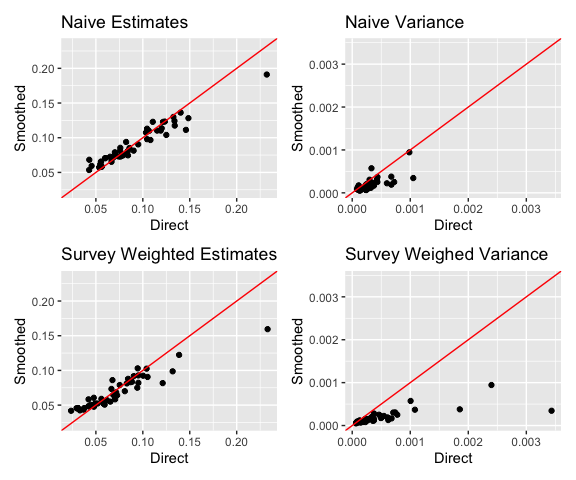### Area-level covariates Covariates at the area level could be included in the smoothing model by specifying the X argument. The first column of the covariate matrix should be the names of regions that match the column and row names of the adjacency matrix. Education <- svyby(~educau, ~hracode, design, svymean) Education <- Education[, 1:5] svysmoothed_with_covariate <- smoothSurvey(data = BRFSS, geo = KingCounty, Amat = mat, X = Education, responseType = "binary", responseVar = "diab2", strataVar = "strata", weightVar = "rwt_llcp", regionVar = "hracode", clusterVar = "~1", CI = 0.95) svysmoothed_with_covariate$fit$summary.fixed ## mean sd 0.025quant 0.5quant 0.97quant mode ## (Intercept) -2.67 0.071 -2.81 -2.67 -2.54 -2.67 ## educauless.than.HS 1.93 3.206 -4.41 1.94 7.95 1.95 ## educauHS.grad -1.36 1.350 -4.02 -1.37 1.19 -1.37 ## educausome.college 1.59 1.144 -0.66 1.59 3.75 1.58 ## educaucollege.grad -0.74 0.507 -1.73 -0.74 0.22 -0.75 ## kld ## (Intercept) 2.9e-07 ## educauless.than.HS 9.1e-07 ## educauHS.grad 1.3e-06 ## educausome.college 5.9e-07 ## educaucollege.grad 5.5e-06 ### Prior specification The smoothSurvey function has some default hyperprior choices built in using the Penalising Complexity (PC) priors. To change the hyperparameters of the PC prior, we can simply use the pc.u and pc.alpha for priors on precision parameters, and pc.u.phi and pc.alpha.phi for mixing parameter $$\phi$$ in the BYM2 model for spatial effects. The interpretation of PC prior parameters for the precision $$\tau$$ is $\mbox{Prob}(\sigma > u) = \alpha$ where $$\sigma = 1 / \sqrt{\tau}$$ is the standard deviation. And for the mixing parameter $$\phi$$ is $\mbox{Prob}(\phi < u) = \alpha$ For example, if we change pc.u and pc.alpha from the default value $$u = 1, \alpha = 0.01$$ to $$u = 0.1, \alpha = 0.01$$, we would assign more prior mass on smaller variance of the random effects. svysmooth.1 <- smoothSurvey(data = BRFSS, geo = KingCounty, Amat = mat, responseType = "binary", responseVar = "diab2", strataVar = NULL, weightVar = NULL, regionVar = "hracode", clusterVar = "~1", CI = 0.95, pc.u = 0.1, pc.alpha = 0.01) We can visualize one or more metrics on the map is by the mapPlot function, for example, toplot <- svysmoothed$smooth
toplot$mean.new <- svysmooth.1$smooth$mean toplot$var.new <- svysmooth.1$smooth$var
variables <- c("mean", "mean.new", "var", "var.new")
names <- c("Mean (default prior)", "Mean (new prior)", "Variance (default prior)",
"Variance (new prior)")
g1 <- mapPlot(data = toplot, geo = KingCounty, variables = variables[1:2],
labels = names[1:2], by.data = "region", by.geo = "HRA2010v2_", size = 0.1)
g2 <- mapPlot(data = toplot, geo = KingCounty, variables = variables[3:4],
labels = names[3:4], by.data = "region", by.geo = "HRA2010v2_", size = 0.1)
g1/g2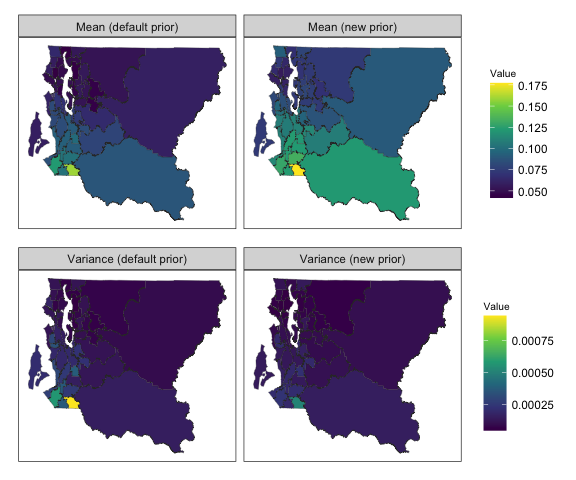A more complex way to change the random effect model entirely is to use the formula argument. To use this option, we will need to use the internal indicator for region, region.struct or region.unstruct as the index. This index variable name can also be observed from the fitted object by summary(svysmoothed$fit) or svysmoothed$formula. For example, we can reassign a different parameterization of the BYM model. For users familiar with INLA syntax, this allows more possibilities of expanding the modeling framework.

 formula <- "f(region.struct, model = 'besag', graph = Amat,
constr = TRUE, scale.model = TRUE) +
f(region.unstruct, model = 'iid')"
svysmooth.2 <- smoothSurvey(data = BRFSS, geo = KingCounty, Amat = mat,
responseType = "binary", responseVar="diab2",
strataVar="strata", weightVar="rwt_llcp", regionVar="hracode",
clusterVar = "~1",   CI = 0.95,
formula = formula)

## Estimating Under-5 Mortality Rates in Space and Time

DemoData contains model survey data provided by DHS. Note that this data is fake, and does not represent any real country’s data. Data similar to the DemoData data used in this vignette can be obtained by using getBirths. DemoMap contains geographic data from the 1995 Uganda Admin 1 regions defined by DHS. Data similar to the DemoMap data used in this vignette can be obtained by using read_shape.

data(DemoData)
data(DemoMap)
geo <- DemoMap$geo mat <- DemoMap$Amat

DemoData is a list of $$5$$ data frames where each row represent one person-month record and contains the $$8$$ variables as shown below. Notice that time variable is turned into 5-year bins from 80-84 to 10-14.

summary(DemoData)
##      Length Class      Mode
## 1999 8      data.frame list
## 2003 8      data.frame list
## 2007 8      data.frame list
## 2011 8      data.frame list
## 2015 8      data.frame list
head(DemoData[])
##   clustid id  region  time  age weights        strata died
## 1       1  1 eastern 00-04    0     1.1 eastern.rural    0
## 2       1  1 eastern 00-04 1-11     1.1 eastern.rural    0
## 3       1  1 eastern 00-04 1-11     1.1 eastern.rural    0
## 4       1  1 eastern 00-04 1-11     1.1 eastern.rural    0
## 5       1  1 eastern 00-04 1-11     1.1 eastern.rural    0
## 6       1  1 eastern 00-04 1-11     1.1 eastern.rural    0

DemoData is obtained by processing the raw DHS birth data (in .dta format) in R. The raw file of birth recodes can be downloaded from the DHS website https://dhsprogram.com/data/Download-Model-Datasets.cfm. For this example dataset, no registration is needed. For real DHS survey datasets, permission to access needs to be registered with DHS directly. DemoData contains a small sample of the observations in this dataset randomly assigned to $$5$$ example DHS surveys.

Here we demonstrate how to split the raw data into person-month format from. Notice that to read the file from early version of stata, the package readstata13 is required. The following script is based on the example dataset ZZBR62FL.DTA available from the DHS website. We use the interaction of v024 and v025 as the strata indicator for the purpose of demonstration. We can see from range(data$v008) that the CMC code for the date of interview corresponds to the year 1900 + floor(1386/12)= 2015. In practice, however, the survey year is usually known. The survey year variable allows the mis-recorded data. Dates after the surveyyear will be removed. Thus for survey taking place over multiple years, the later year is suggested to be used as surveyyear. If set to NA then no checking will be performed. library(readstata13) my_fp <- "data/ZZBR62DT/ZZBR62FL.DTA" dat <- getBirths(filepath = my_fp, surveyyear = 2015, strata = c("v024", "v025")) dat <- dat[, c("v001", "v002", "v024", "per5", "ageGrpD", "v005", "strata", "died")] colnames(dat) <- c("clustid", "id", "region", "time", "age", "weights", "strata", "died") ### Horvitz-Thompson estimators of U5MR Next, we obtain Horvitz-Thompson estimators using getDirectList. We specify the survey design as the two-stage stratified cluster sampling where strata are specified in the strata column, and clusters are specified by both the cluster ID (clusterid) and household ID (id). years <- levels(DemoData[]$time)
data_multi <- getDirectList(births = DemoData, years = years, regionVar = "region",
timeVar = "time", clusterVar = "~clustid+id", ageVar = "age", weightsVar = "weights",
geo.recode = NULL)

Before fitting the model, we also aggregate estimates based on different surveys into a single set of estimates, using the inverse design-based variances as the weights.

dim(data_multi)
##  150  11
data <- aggregateSurvey(data_multi)
dim(data)
##  30 10

### National estimates of U5MR

With the combined direct estimates, we are ready to fit the smoothing models. First, we ignore the subnational estimates, and fit a model with temporal random effects only. In this part, we use the subset of data region variable being “All”. In fitting this model, we first define the list of time periods we wish to project the estimates on. First we can fit a Random Walk 2 only model defined on the 5-year period.

years.all <- c(years, "15-19")
fit1 <- smoothDirect(data = data, Amat = NULL, year_label = years.all,
year_range = c(1985, 2019), time.model = "rw2", is.yearly = FALSE,
m = 5)
## ----------------------------------
## Smoothed Direct Model
##   Main temporal model:        rw2
##   Temporal resolution:        period model (m = 1)
## ----------------------------------

We can also estimate the Random Walk 2 random effects on the yearly scale, with direct estimates calculated in 5-year intervals.

fit2 <- smoothDirect(data = data, Amat = NULL, year_label = years.all,
year_range = c(1985, 2019), time.model = "rw2", is.yearly = TRUE, m = 5,
type.st = 4)
## ----------------------------------
## Smoothed Direct Model
##   Main temporal model:        rw2
##   Temporal resolution:        yearly model (m = 5)
## ----------------------------------

The marginal posteriors are already stored in the fitted object. We use the following function to extract and re-arrange them.

out1 <- getSmoothed(fit1)
out2 <- getSmoothed(fit2)

We can compare the results visually using the function below.

g1 <- plot(out1, is.subnational = FALSE) + ggtitle("National period model")
g2 <- plot(out2, is.subnational = FALSE) + ggtitle("National yearly model")
g1 + g2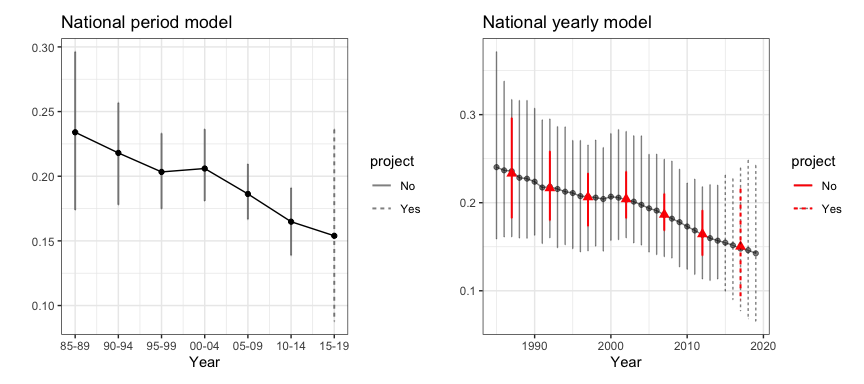### Subnational estimates of U5MR

Now we fit the full model on all subnational regions. First, we use the Random Walk 2 model defined on the 5-year period.

fit3 <- smoothDirect(data = data, Amat = mat, year_label = years.all, year_range = c(1985,
2019), time.model = "rw2", is.yearly = FALSE, type.st = 4)
## ----------------------------------
## Smoothed Direct Model
##   Main temporal model:        rw2
##   Temporal resolution:        period model (m = 1)
##   Spatial effect:             bym2
##   Interaction temporal model: rw2
##   Interaction type:           4
## ----------------------------------
out3 <- getSmoothed(fit3)

Similarly we can also estimate the Random Walk 2 random effects on the yearly scale.

fit4 <- smoothDirect(data = data, Amat = mat, year_label = years.all, year_range = c(1985,
2019), time.model = "rw2", is.yearly = TRUE, m = 5, type.st = 4)
## ----------------------------------
## Smoothed Direct Model
##   Main temporal model:        rw2
##   Temporal resolution:        yearly model (m = 5)
##   Spatial effect:             bym2
##   Interaction temporal model: rw2
##   Interaction type:           4
## ----------------------------------
out4 <- getSmoothed(fit4)

The figures below shows the comparison of the subnational model with different temporal scales.

g1 <- plot(out3, is.yearly = FALSE, is.subnational = TRUE) + ggtitle("Subnational period model")
g2 <- plot(out4, is.yearly = TRUE, is.subnational = TRUE) + ggtitle("Subnational yearly model")
g1 + g2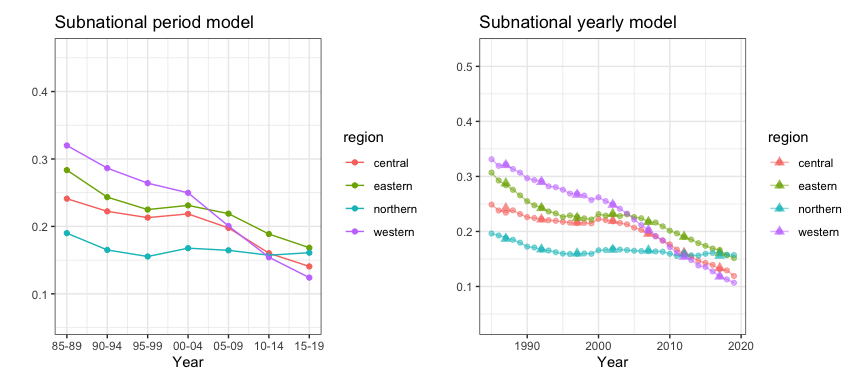We can also add back the direct estimates for comparison when plotting the smoothed estimates.

plot(out4, is.yearly = TRUE, is.subnational = TRUE, data.add = data_multi,
option.add = list(point = "mean", by = "surveyYears")) + facet_wrap(~region,
scales = "free")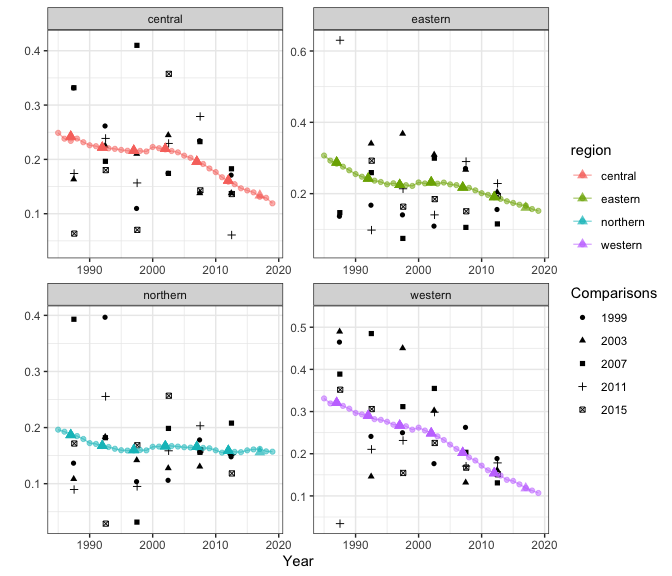Finally, we show the estimates over time on maps.

mapPlot(data = subset(out4, is.yearly == F), geo = DemoMap\$geo, variables = c("years"),
values = c("median"), by.data = "region", by.geo = "NAME_final", is.long = TRUE,
ncol = 4)